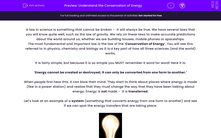# Understand the Conservation of Energy

In this worksheet, students will revise the law of energy conservation and energy transfer. They will use the law of conservation to calculate efficiency.Key stage:  KS 4

Year:  GCSE

GCSE Boards:   AQA, AQA Trilogy, AQA Synergy, OCR 21st Century, OCR Gateway, Pearson Edexcel, Eduqas,

Popular topics:   Biology worksheets, Physics worksheets, Chemistry worksheets,

Difficulty level:#### Worksheet Overview

A law in science is something that cannot be broken -  it will always be true. We have several laws that you will know quite well, such as the law of gravity. We rely on these laws to make accurate predictions about the world around us, whether we are building houses, mobile phones or spaceships.

The most fundamental and important law is the law of the ‘Conservation of Energy’. You will see this referred to in physics, chemistry and biology as it is a key part of how all three sciences (and the world) works.

It is fairly simple, but because it is so simple you MUST remember it word for word! Here it is:

‘Energy cannot be created or destroyed; it can only be converted from one form to another.’

When people first hear this, it can blow their mind. They start to think about places where energy is made (like in a power station) and realise that they must change the way that they have been talking about energy. Energy is not made -  it is transferred.

Let’s look at an example of a system (something that converts energy from one form to another) and see if we can spot the energy transfers that are taking place.Let's take this light bulb.

What energy is going into it? Electrical.

The energy store that is depleting is the electrical store. This is because the electrical energy is being converted into light and heat energy. We want the light energy as this is useful.  However, we also get some wasted energy in the form of heat. We didn’t design a light bulb to get hot, but it does. This is what we call wasted energy as it is not doing the job that we want it to do.

When we compare the amount of energy we want with the amount of energy we don’t want, we come up with a number which is called its efficiency. Efficiency is a simple measurement telling us how much of the energy is going into doing the job that we have designed it for. It can be presented as a number between 0 and 1 (where 0 is none of the energy going into what we want it for, and 1 is where all of the energy is going into what we want it for) or as a percentage.

FUN FACT – it is impossible to get a system that has an efficacy of 100%. Not just difficult, not just that we have never managed to achieve it, but it's impossible! It's another one of those laws.

Let's look at how you work out efficiency:Here's an example:

A light bulb takes in 100 joules (J) of energy every second. 40 J of that energy are given off as light. Calculate the efficiency of the light bulb.

Step 1  Highlight all of the numbers in the question.

A light bulb takes in 100 J of energy every second. 40 J of that energy are given off as light. Calculate the efficiency of the light bulb.

Step 2  Find out what each value is.

Energy in = 100 J

Useful energy out = 40 J

Step 3  Put the number into the equation.

Efficiency = 40 ÷ 100

Step 4  Press = and get your number (if it asks for a percentage, all you need to do is x 100 at the very end).

Efficiency  = 0.4 or 40%

There are no units for efficiency, but if it is a percentage, then always put the percentage sign at the end.

Are you ready for some questions now?

### What is EdPlace?

We're your National Curriculum aligned online education content provider helping each child succeed in English, maths and science from year 1 to GCSE. With an EdPlace account you’ll be able to track and measure progress, helping each child achieve their best. We build confidence and attainment by personalising each child’s learning at a level that suits them.

Get started